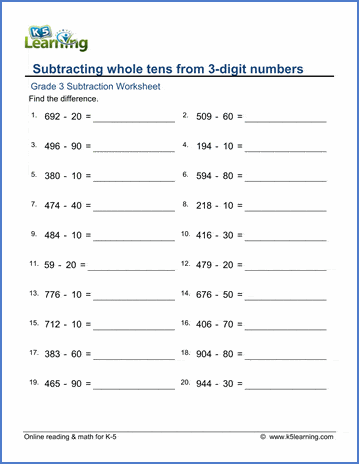# 3rd Grade Math Worksheets: Subtraction

## Subtraction worksheets for grade 3

Our 3rd grade subtraction worksheets include both mental subtraction and column form subtraction.

## Mental Subtraction

Example

Subtract a 1-digit number from a 2-digit number   54 − 8 =  __
Subtract a 1-digit number from a 2-digit number - missing number
54 - ___ =  46

Subtract whole tens from a 2-digit number   83 − 30 =
Subtract whole tens from a 2-digit number - missing number   83 - ___ = 53
Subtract whole tens from a 3-digit number   222 − 50 =
Subtract whole tens from a 3-digit number - missing number   ___ - 50 = 172
Subtract whole hundreds from a 3-digit number   899 − 800 =

Subtract a 2-digit number from whole hundreds   300 − 77 =
Subtract whole hundreds from whole hundreds   1,900 − 600 =
Subtract whole hundreds from 4-digit numbers   5,962 − 600 =
1,000 − 240 =
4,000 − 821 =
Subtract a 3-digit number from any whole thousand - missing number   4,000 - ___ = 3,179

872

- 542

5,122

- 2,014

600

- 89

3,000

- 131

## Subtraction word problems